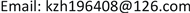﻿ 关于极限环判断的一个注记 A Note on the Judgment of Limit Cycles

Pure Mathematics
Vol.07 No.06(2017), Article ID:22675,3 pages
10.12677/PM.2017.76060

A Note on the Judgment of Limit Cycles

Zhihong Kong

Department of Mathematics, Taiyuan Normal University, Jinzhong ShanxiReceived: Oct. 28th, 2017; accepted: Nov. 8th, 2017; published: Nov. 15th, 2017ABSTRACT

Isolation in isolated periodic solutions implies that the system neither exists other periodic solutions nor exists singular points of the system in its neighborhood. We give examples to illustrate that the closed trajectories in an annular region are not all limit cycles, although the right-side functions are analytic functions. In our case, there are singular points in the periodic solution.

Keywords:Analytic Vector Field, Annular Region, Limit Cycles, Singular Points, Isolated Periodic Solutions1. 引言

Poincaré-Bendixson环域定理  说明了，只要能构造出一个有界的环形闭域D，在其上沿有奇点，且在其边界上轨线均进入(或离开)该域，自然，进入(或离开)该域的解均不会再离开(或进入)域D，那么可以肯定在域D内必存在周期解(闭轨线)。如果这周期解(闭轨线)是孤立的，那么它就是极限环，这样，可以通过构造特殊的环域来寻求极限环，并大致确定其位置，如果环域越狭小，则极限环的位置就越准确。

$\frac{\text{d}x}{\text{d}t}=X\left(x,y\right),\text{\hspace{0.17em}}\text{\hspace{0.17em}}\frac{\text{d}y}{\text{d}t}=Y\left(x,y\right)$ (1)

2. 例子与相关结果

$\left\{\begin{array}{l}\frac{\text{d}x}{\text{d}t}=x\left({x}^{2}+{y}^{2}-1\right)\left({x}^{2}+{y}^{2}-9\right)-y\left({x}^{2}+{y}^{2}-2x-8\right)\\ \frac{\text{d}y}{\text{d}t}=y\left({x}^{2}+{y}^{2}-1\right)\left({x}^{2}+{y}^{2}-9\right)+x\left({x}^{2}-{y}^{2}-2x-8\right)\end{array}$ (2)

$\left\{\begin{array}{l}\frac{\text{d}r}{\text{d}t}=r\left({r}^{2}-1\right)\left({r}^{2}-9\right)\\ \frac{\text{d}\theta }{\text{d}t}={r}^{2}-2r\mathrm{cos}\theta -8\end{array}$ (3)

$r<1$$\frac{\text{d}r}{\text{d}t}=r\left({r}^{2}-1\right)\left({r}^{2}-9\right)>0$ ，r单调增加， $\frac{\text{d}\theta }{\text{d}t}={r}^{2}-2r\mathrm{cos}\theta -8$${r}^{2}-2r\mathrm{cos}\theta$ 的最大值为 ${r}^{2}+2r$$\frac{\text{d}\theta }{\text{d}t}<{r}^{2}+2r-8={\left(r+1\right)}^{2}-9<4-9=-5<0$$\theta$ 单调减少；当 $1$\frac{\text{d}r}{\text{d}t}=r\left({r}^{2}-1\right)\left({r}^{2}-9\right)<0$ ，r单调减少，同样， ${r}^{2}-2r\mathrm{cos}\theta$ 的最大值为 ${r}^{2}+2r$ ，此时 $\frac{\text{d}\theta }{\text{d}t}={r}^{2}+2r-8={\left(r+1\right)}^{2}-9<9-9=0$$\theta$ 单调减少；当 $2 时， $\frac{\text{d}r}{\text{d}t}=r\left({r}^{2}-1\right)\left({r}^{2}-9\right)<0$ ，r单调减少， $\mathrm{max}\left(\frac{\text{d}\theta }{\text{d}t}\right)={\left(r+1\right)}^{2}-9>0$$\mathrm{min}\left(\frac{\text{d}\theta }{\text{d}t}\right)={r}^{2}-2r-8={\left(r-1\right)}^{2}-9<0$$\frac{\text{d}\theta }{\text{d}t}$ 变号；当 $r>3$$\frac{\text{d}r}{\text{d}t}=r\left({r}^{2}-1\right)\left({r}^{2}-9\right)>0$ ，r单调增加， $\frac{\text{d}\theta }{\text{d}t}$ 变号。

$\left\{\begin{array}{l}{x}^{2}+{y}^{2}-9=0\\ {x}^{2}+{y}^{2}-2x-8=0\end{array}$ (4)

$\left\{\begin{array}{l}\frac{\text{d}x}{\text{d}t}=x\left({x}^{2}+{y}^{2}-1\right)\left({x}^{2}+{y}^{2}-n\right)-y\left({x}^{2}+{y}^{2}-2x-n+1\right)\\ \frac{\text{d}y}{\text{d}t}=y\left({x}^{2}+{y}^{2}-1\right)\left({x}^{2}+{y}^{2}-n\right)+x\left({x}^{2}+{y}^{2}-2x-n+1\right)\end{array}$ (5)

$\left\{\begin{array}{l}\frac{\text{d}x}{\text{d}t}=x\left({x}^{2}+{y}^{2}-1\right)\left({x}^{2}+{y}^{2}-n\right)-y\left({x}^{2}+{y}^{2}-2y-n+1\right)\\ \frac{\text{d}y}{\text{d}t}=y\left({x}^{2}+{y}^{2}-1\right)\left({x}^{2}+{y}^{2}-n\right)+x\left({x}^{2}+{y}^{2}-2y-n+1\right)\end{array}$ (6)

3. 结语

A Note on the Judgment of Limit Cycles[J]. 理论数学, 2017, 07(06): 461-463. http://dx.doi.org/10.12677/PM.2017.76060

1. 1. 丁同仁, 李承治. 常微分方程教程[M]. 第2版. 北京: 高等教育出版社, 2004: 270-272.

2. 2. 王高雄, 周之铭, 朱思铭, 等. 常微分方程[M]. 第3版. 北京: 高等教育出版社, 2006: 295-297.